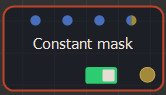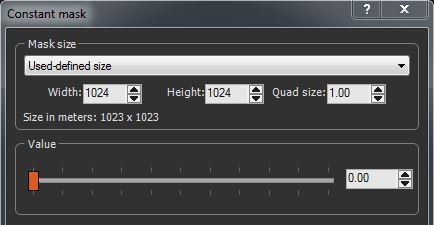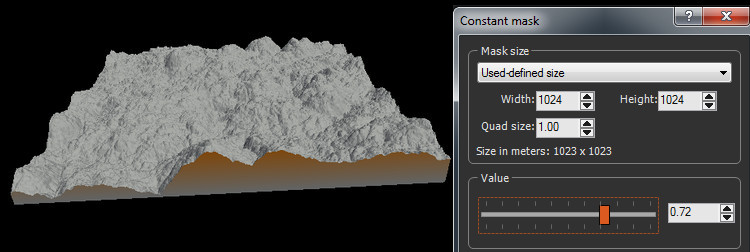This node creates a mask with a set size and a constant value.Double click on the node to open its parameters:### Editing a Constant mask node

• Mask size: Edit the mask's Width, Height, and Quad size, or use a size preset from the list.
• Use the Value slider to set the constant value of the mask: a value of 0 = black mask; a value of 1 = white mask### Parameters

Parameter Use
Mask size Sets the mask's Width, Height, and Quad size or use a size preset from the list.
Value Sets the constant value of the mask: a value of 0 = black mask; a value of 1 = white mask